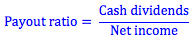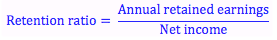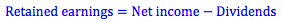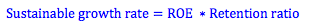Explore BrainMass

# Payout, Retention and Growth Ratios

The payout ratio is the proportion of net income after interest and taxes that the firm pays out in dividends. The retention ratio is opposite: the proportion of net income that is kept as retained earnings. Retained earnings is equal to a firm’s net income less the amount of dividends the firm pays.The retention ratio may also be called the plowback ratio. It is important because it shows us how much of the firm’s income it keeps to reinvest in future growth of the firm. From this, we can calculate what we call the sustainable growth rate. This is the rate of growth implied by the retention ratio and the firm’s current return on equity. That is, a firm should grow at a rate equal to the amount of earnings it retains each year multiplied by the return it expects from these earnings.This tells us the rate of growth we can expect from the firm at the current level of dividends and without increasing financial leverage. This growth rate, g, can be used in the dividend discount model for stock valuation.